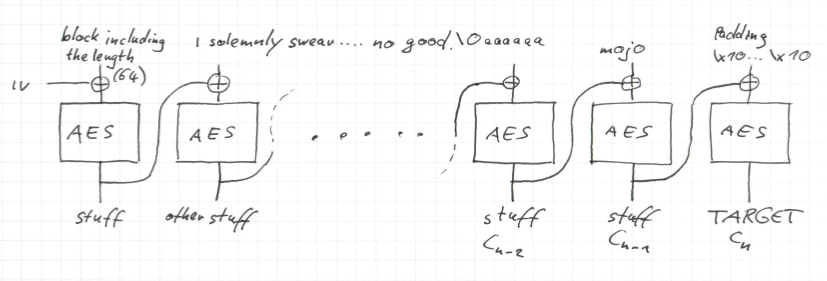## Intro

From 2016-09-30 to 2016-10-02 i, together with the Ulm Security Sparrows took part in the TUM-CTF organized by the team h4x0rpsch0rr from TU Munich. As usual in CTFs there were some challenges and if you solved one correctly a special flag in form of a binary string appears from somewhere. This flag can be submitted in the web-interface and your team gets points.

This post contains my part of the writeup. For the full writeup please visit the USS-Blog.

## Haggis

The Haggis challenge started with the following greeting:

OMG, I forgot to pierce my haggis’ sheep stomach before cooking it, so

(Fun fact: Haggis hurling is a thing.)

nc $IP$PORT


Connecting to the port yielded some hex-code. There was also a file attached with the following content:

This was most probably the code running on the server. Let’s look at it step by step. There is a padding defined which takes a message as input and pads it such that its length is a multiple of 16 Bytes. If one byte is missing, it adds a one, if two bytes are missing it adds two twos. If three bytes are missing it adds three threes. And so on. If the message is already a multiple of 16 Bytes, it adds 16 16s to the end. So there is one whole block of padding at the end.

The function haggis takes a messages and computes some kind of CBC-MAC. It prepends the length of the message. This length fills one whole block. Then comes the message itself together with the padding to make the length a multiple of the block size of the cipher. All of this is encrypted with the AES-cipher in CBC mode. The key used is 0x10, the initialization vector is set to zero. This is not in the code, but it can be found in the documentation that this is the default. The function haggis returns the last encrypted block. This block depends on all of the previous blocks because that is the way CBC works.

The code then chooses a random target, which is encoded in hex and printed. It waits for an incoming msg which is hex-decoded. If msg starts with the string ‘I solemnly swear that I am up to no good.\0’ and if haggis(m) yields the target then the flag is printed.

So our task is pretty clear: For a given target we need to find a msg where haggis(msg)==target and msg needs to start with ‘I solemnly swear that I am up to no good.\0’.

To solve this challenge, remember how CBC works. CBC splits the input in blocks $$P_i$$ of equal length and computes $$C_i=E(C_{i-1}\oplus P_i)$$, where $$\oplus$$ is the xor operator. $$C_0$$ by the way is the initialization vector. We can decrypt a single block, since we know the key. It was just 0x10. To make things easier, we restrict some variables such that we have fewer choices to make. We know that our msg needs to start with ‘I solemnly swear that I am up to no good.\0’. Let us append ‘aaaaaa’ to make this a multiple of the block size. Next comes a block further referred to as mojo which we will choose in a very special way. The final block consists of the padding. It is one block full of padding, so in Python it looks like this: b’\x10\x10\x10\x10\x10\x10\x10\x10\x10\x10\x10\x10\x10\x10\x10\x10’. Then we already know the length of the whole plaintext, namely 64 Bytes which we can prepend. All in all, we get the arrangement shown in the image.Note the only part of the plaintext which is not fixed is the block containing the mojo. This mojo contains the whole magic of the forgery of the MAC. Regarding the ciphertext for the most part we do not care, except for the last block. This should be the target we receives from the server. So we can consider it as fixed.

How can we find a suitable mojo? We can trace the target backwards through the CBC. For sake of notation, let $$n$$ denote the last block, so $$C_n=target$$. We can decrypt $$C_n$$ such that we end up on top of the last block. Xoring with the padding yields $$C_{n-1}$$. If we decrypt $$C_{n-1}$$ with a single round of AES and xor it with $$C_{n-2}$$ then we get the mojo. But what is $$C_{n-2}$$? To compute this, we can start from the left hand side and encrypt with CBC. It happens to be the CBC encryption of the blocks up to then, i.e., the encryption of the string ‘I solemnly swear that I am up to no good.\0aaaaaa’. And that is already everything.

There were some programming issues with AES objects in CBC-mode being stateful, which we did not expect and was a source of confusion. We did not talk to the IP with Python, but instead copied the given target from Netcat into an ipython. There we computed the dehaggised target and copied it into our NetCat process. This yielded the flag.

05 November 2016

Writeup